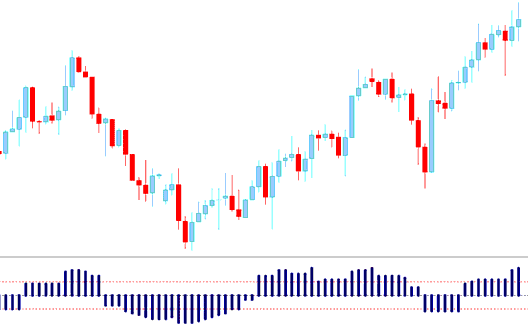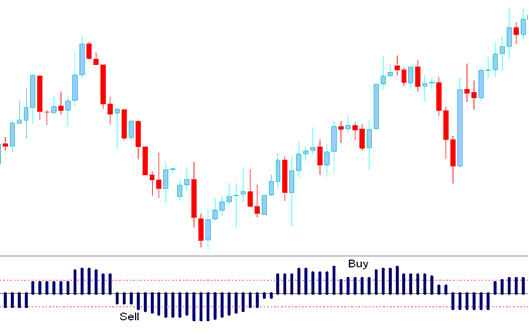## Aroon Oscillator Forex Technical Analysis and Aroon Oscillator Forex Trading Signals

Calculated from Aroon Indicator.

Calculation

The oscillator is calculated by subtracting Aroon DOWN from Aroon UP.

The Oscillator oscillates between -100 and +100, this is because both Aroon UP and Aroon DOWN moves between Zero and 100.## Forex Technical Analysis and Generating Forex Trading Signals

The Oscillator gives a technical buy signal when it goes above the Zero line. The further it is from zero the stronger the up trend

The indicator shows price is gaining momentum if it keeps moving towards +100, and momentum is reducing if it is approaching the Zero mark.

## Sell Signal

The Oscillator gives a technical short signal when it goes below the Zero line. The further it is from zero the stronger the down trend

The indicator shows price is gaining momentum if it keeps moving towards -100, and momentum is reducing if it is approaching the Zero mark.Aroon Oscillator and Sell Forex Trading Signals - How to Analyze Aroon Oscillator Forex Indicator